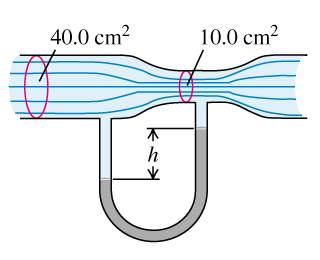# Difference in height in a U-shaped tube

## Homework Statement

The horizontal pipe, shown in the figure, has a cross-sectional area of $40,0 cm^{2}$ at the wider portions and $10,0 cm^{2}$ at the constriction. Water is flowing in the pipe, and the discharge from the pipe is $6,00*10^{-3}\frac{m^{3}}{s} (6,00\frac{L}{s})$. The density of mercury is $\rho_{Hg}=13,6*10^{3}\frac{kg}{m^{3}}$ and the density of water is $\rho_{w}=1,00*10^{3}\frac{kg}{m^{3}}$.

What is the difference in height between the mercury columns in the U-shaped tube?## Homework Equations

I've been using Bernoulli's Principle.

$P_{1}+\rho*g*H_{1}=P_{2}+\rho*g*H_{2}$

## The Attempt at a Solution

When I use the formula above I get 0.127 m which is incorrect. I also found this problem on the internet where they get the same answer so I was pretty sure but apparently it's wrong. :(

Last edited:

Where is your picture? I suspect one end of the manometer attached to the smaller diameter while the other is sampling the larger diameter. If so there is a difference in velocity so the pressure must change. You'll probably need some V^2 terms in your equation to account for velocity differences. We need a picture of your problem.

SteamKing
Staff Emeritus
Homework Helper
You have the density of water and the density of mercury as roughly equal.

Sorry! I've put in the picture and the correct density of water. :)

OK I see your picture now. Don't know where it was before because it was not showing up on my screen. Because the velocity changes you must have a V^2 on each side of the equation. You compute the velocities from the cross sectional areas and flow rate.

Should I use $A_{1}v_{1}=A_{2}v_{2}$? A and v are the areas and velocities. I can use $A_{1}$ and $v_{1}$ for the wide part... then I can find $v_{2}$. Does that help?

Okay my calculations are $A_{1}v_{1}=40 cm^{2}*6,00\frac{L}{s}=240\frac{L*cm^{2}}{s}$. Then I get $A_{2}v_{2}=10 cm^{2}*v_{2}=240\frac{L*cm^{2}}{s}$ so $v_{2}=24\frac{L}{s}$.

Your units are liters per second. That is not the unit of velocity. You need meters/second. V1 is not 6 liters/second.

Always check to ensure your units describe the variable you seek.

Last edited: